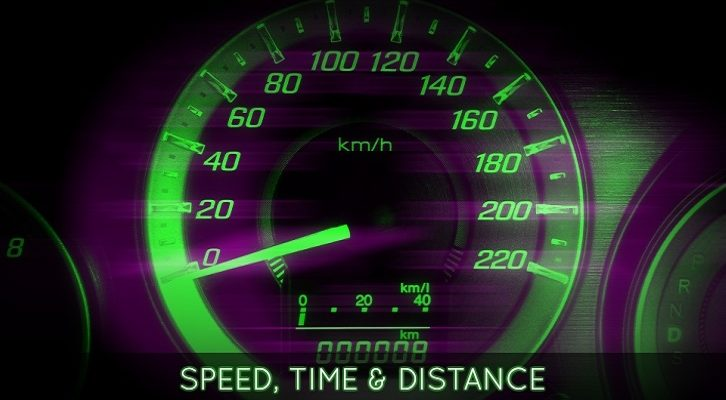# Races – Basic Questions

Have given below a few basic questions in Races

1. In a 100m race, A can give B a start of 12 meters or 3 seconds. What is the speed of B in m/sec and how long will A take to run 100m?

2. A can give B a start of 30 in a km race and A can give C a start of 50m in a km race. How much start will B be able to give C in a km race?

3. A runs a 400m race at the speed of 8 m/sec. He gives B a start of 40m and still beats him by 10 seconds. What is B’s speed?

4. If A gives B a start of 20m in a race, A beats him by 10 seconds. On the contrary, if A gives B a start of 40m, they finish the race in a dead heat. If the length of the race is 400m, how long will A take to run the race?

5. A and B start around a circular track of length 600m at speeds of 6m/s and 9 m/sec from the same point, simultaneously and in the same direction. When will they meet for the first time? How far from the starting point will they meet, when they meet for the first time?

6. A and B run around a circular track at speeds of 8m/sec and 5m/sec starting from the same point, simultaneously and in the same direction. How many points with respect to the starting point on the circular track will they meet, if the run at the above mentioned constant speeds endlessly?